ISSAC 96 - Hypergeometric Function Representations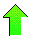Contents News View

 Contiguity Relations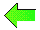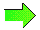Let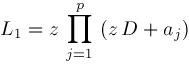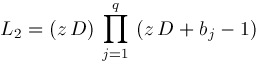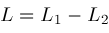The differential equation for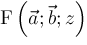becomes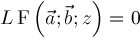Now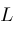is a polynomial in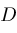but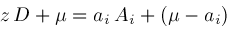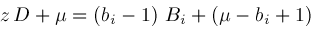socan also be expressed as a polynomial in terms of shift operators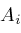and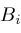converting the differential equation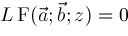into a difference equation among contiguous instances of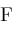which we call a contiguity relation.  Operators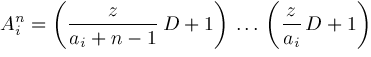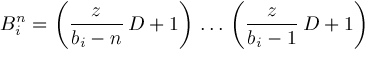are defined if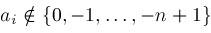and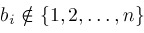respectively.

If we expressas a polynomial in, then we get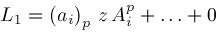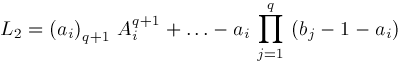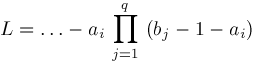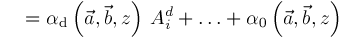which has degree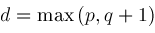If we expressas a polynomial in, we get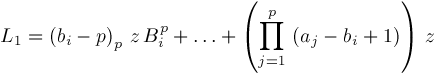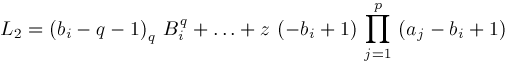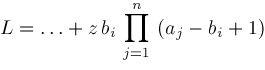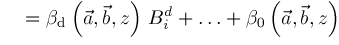which has degree at mostThese results let us define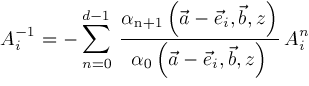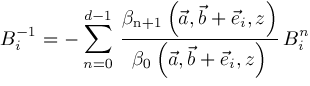The coefficients of these polynomials inandare defined when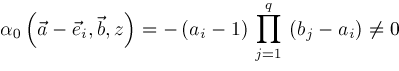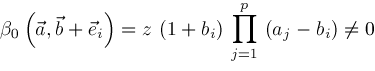Operators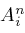and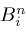for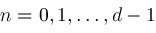are defined if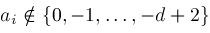and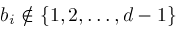respectively.  Hence,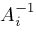is defined if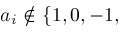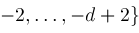and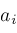is distinct from all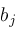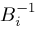is defined ifand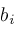is distinct from all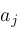.  Recall that